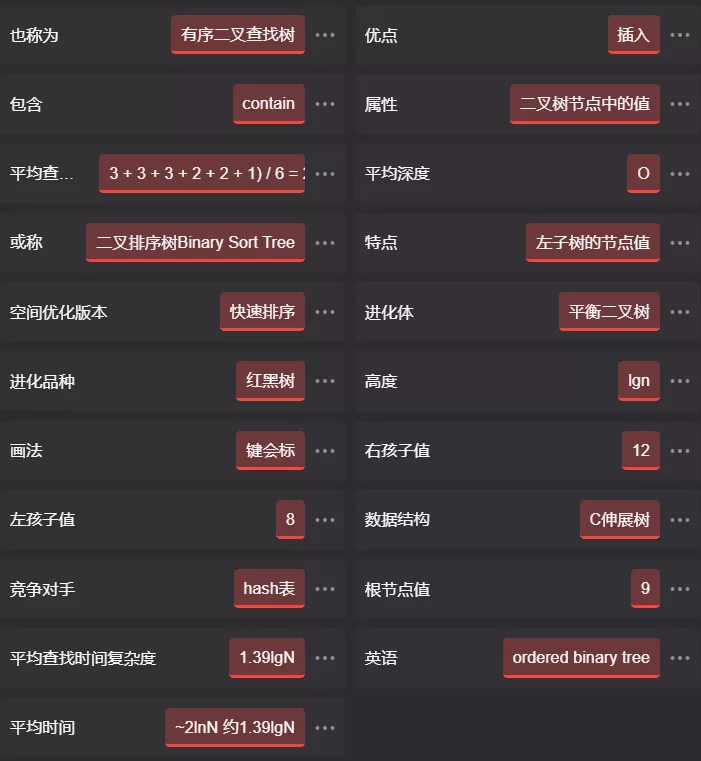+关注继续查看

# 问题分析：

1. 把 A 平均分为前后两个部分，前部分有 x 个元素，后部分有 n-x 个元素

（由于 A 是有序的，所以后一部分的所有元素大于前一部分）。A[x] = A的

2. 同理把 B 也平均分成前后两个部分，前部分有 y 个元素，后部分有 m-y 个元素。
B[y] = B的后一部分的第一个元素。

3. 由于两个数组都是被平均分割的，所以可以近似地认为 x = n/2, y = m/2。

# part2:

B[y] 及其后面有 m-y 个元素。那么，由于 A[x] <= B[y]，所以合并起来之后，
B[y] 后面那些元素必然也在 A[x] 后面，则合并后 A[x] 后面至少有
(n-x-1) + (m-y) = (n+m)-(x+y+1) 个元素。

# all:

1. A没有了，这样只需要找出 B 中第 k 大的元素，也就是 B[k].
2. B没有了，同上结果就是 A[k].

[c-sharp] view plaincopy
1. /************************************************************************
2.  * This is the practice1 of the Algorithms It solved the problem1 below:
3.  *
4.  * Give a divide and conquer algorithm for the following problem:
5.  * you are given two sorted lists of size m and n, and are allowed
6.  * unit time access to the ith element of each list. Give an O(lg m + lgn)
7.  * time algorithm for computing the kth largest element in the union of the
8.  * two lists. (For simplicity, you can assume that the elements of the
9.  * two lists are distinct).
10.  *
11.  * The idea of the Algorithm in the help file idea.txt!!
12.  *
13.  * The Algorithm is designed by:Nanne
14.  *
15.  ************************************************************************/
16. #include <iostream>
17. using std::cin;
18. using std::cout;
19. using std::endl;
20. int FindTheKth(int a[],int b[],int aLeft, int aRight, int bLeft, int bRight, int k);
21.
22. int main(){
23.     int sizeA,sizeB;
24.     int Kth;
25.     cout << "AĴС";
26.     cin >> sizeA;
27.     int *arrA = new int[sizeA];
28.     cout << "" << sizeA << "" << endl;
29.     for (int i = 0; i < sizeA; i++)
30.         cin >> arrA[i];
31.     cout << "BĴС";
32.     cin >> sizeB;
33.     int *arrB = new int[sizeB];
34.     cout << "" << sizeB << "" << endl;
35.     for (int i = 0; i < sizeB; i++)
36.         cin >> arrB[i];
37.     while(true){
38.         cout << "õڼλ" << endl
39.             << "λҪ" << sizeA + sizeB << "(-1Ƴ):";
40.         cin >> Kth;
41.         if( Kth != -1){
42.             int res = FindTheKth(arrA,arrB,0, sizeA - 1, 0, sizeB - 1, Kth);
43.             if(res != -1)
44.                 cout << "" << Kth << "λǣ" << res << endl;
45.         }
46.         else
47.             return 0;
48.     }
49. }
50.
51. int FindTheKth(int a[],int b[],int aLeft, int aRight, int bLeft, int bRight, int k) {
52.     int aMid = (aLeft + aRight) / 2, bMid = (bLeft + bRight) / 2;
53.     if (aLeft > aRight) return b[bLeft+k-1];
54.     if (bLeft > bRight) return a[aLeft+k-1];
55.     if (a[aMid] <= b[bMid]) {
56.         if (k <= (aMid - aLeft) + (bMid - bLeft) + 1) {
57.             return FindTheKth(a,b,aLeft, aRight, bLeft, bMid-1, k);
58.         } else {
59.             return FindTheKth(a,b,aMid+1, aRight, bLeft, bRight, k-(aMid-aLeft)-1);
60.         }
61.     } else {
62.         if (k <= (aMid - aLeft) + (bMid - bLeft) + 1) {
63.             return FindTheKth(a,b,aLeft, aMid-1, bLeft, bRight, k);
64.         } else {
65.             return FindTheKth(a,b,aLeft, aRight, bMid+1, bRight, k-(bMid-bLeft)-1);
66.         }
67.     }
68.     return -1;
69. }876 0js实现二分查找

638 0846 0624 0733 04519 07830 0589 0494 0linux下练习 c++ 二分查找
#include using namespace std; class person { string name; int age; public: person(const char* n,int a):name(n),age(a){} friend bool operator b.
631 0

761

0

《SaaS模式云原生数据仓库应用场景实践》

《看见新力量：二》电子书